# Testwork: Bond ball mill work index

## Testwork: Bond Ball Mill Work Index

The Bond ball mill work index is one of the most commonly used grindability tests in mining, and is often referred to as the Bond work index.

The test is a 'locked-cycle' test where ground product is removed from test cycles and replaced by fresh feed. The test much achieve a steady-state before completion.

### Sample Requirements

The test requires about 8 kg of material. Although it can work on feed as fine as 2.5 mm, it is best to send material to the testing laboratory that is nominally at least 8 mm (including the natural fines that are part of the sample). The laboratories have a standard way of reducing the coarse material to the (roughly) 2.5 mm size used to feed the test that will not introduce excessive fines.

### Test Inputs

It is necessary for the engineer to specify the desired product size of the test so that the laboratory can choose the appropriate closing mesh screen for conducting the test. This product size is typically the feed size to flotation or leaching. Example product sizes are 200 µm for copper porphyries (select a 212 µm screen), 100 µm for gold cyanidation (select a 150 µm or 125 µm closing screen) or 75 µm for complex sulphides (select a 105 µm screen).

### Test Outputs

The laboratory will report the following information:

• umClosing: The closing mesh size the test was run at, also called P100 or P1. Convert to µm, if needed, before entering into the database.
• F80: Sample feed size in µm (and usually will provide a particle size distribution)
• P80: Sample finished product size in µm (and usually will provide a particle size distribution)
• gpr: The average grams per revolution of the last three cycles (sometimes is labelled GPB)
• fdpassing: The percentage (0-100) of the feed that already passes the closing screen size
• WiBM: The calculated work index (SAGMILLING.COM uses only metric units; if the laboratory reported work index in "short ton" units, multiply that value by 1.1023 and enter the result).

When entering results into the SAGMILLING.COM database table, the following extra fields are available:

• ModBWI: is this test modified from the original Bond protocol? An open-circuit Minnovex ModBWI test or a SAGDesign ball mill work index with non-standard feed would be entered as "true" (or "1" in a batch upload of test results).
• synthetic indicates whether this is a real test result, or just a synthetic one that should only be used for modelling. If this column contains a value of '1' (boolean=true) for a test, then that test is understood to not be a real test result and is therefore not shown on the testwork comparison charts. Synthetic values are available when running circuit model simulations and do show up in the list of model results.

The computation of a Bond ball mill work index was empirically calibrated by Fred Bond using a short ton basis. The modern (metric tonne) basis is the following equation: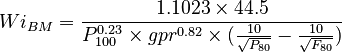$Wi_{BM} = \frac{1.1023 \times 44.5}{P_{100}^{0.23} \times gpr^{0.82} \times (\frac{10}{\sqrt{P_{80}}} - \frac{10}{\sqrt{F_{80}}})}$

NI43-101 reports often provide P100 and the work index. To estimate the other parameters, you can assume:

• the P80 is 0.92 × (P100, µm)0.96 (see below for details)
• assume the F80 is 2440 µm (see Nikolić & Doll paper in preparation)

The grams per revolution can be computed by rearranging Bond's laboratory equation: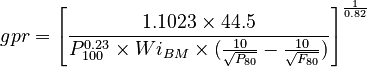$gpr = \left[ \frac{1.1023 \times 44.5}{P_{100}^{0.23} \times Wi_{BM} \times (\frac{10}{\sqrt{P_{80}}} - \frac{10}{\sqrt{F_{80}}})} \right]^\frac{1}{0.82}$

### Derived values composed from WiBM results

• Morrell Mib value is calculated for a sample if the following are available: umClosing, F80, P80, gpr.
• Units are kWh/t based on Morrell's empirical calibration.
• This value is used in the Morrell Mi models.
•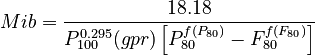$Mib = \frac{18.18}{P_{100}^{0.295} × (gpr) \left [ P_{80}^{f(P_{80})} - F_{80}^{f(F_{80})} \right ]}$ kWh/t
• Levin B parameter is calculated for a sample if the following are available: umClosing, F80, P80, gpr, fdpassing.
• Units of the Levin B are mWh per revolution of a standard Bond grindability ball mill (mWh/rev). Watch out as this metric is also given as kWh/rev in literature.
• This value is not used in any computations, but can be used in external calculation such as Functional Performance.
•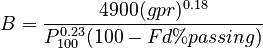$B = \frac{4900 × (gpr)^{0.18}}{P_{100}^{0.23} (100 - Fd%passing)}$ mWh/rev

### Modelling

Ball mill work index is used in several SAGMILLING.COM circuit models, including:

It is also used in the CEET2 model (distributed by SGS) and is commonly used by people running the JK SimMet population balance model (distributed by JK Tech).

The work index is used to calculate the energy requirement to grind rocks in the fine size range, below 2.5 mm into the range of a few hundreds of micrometres. Heterogeneous ore types typically are sensitive to the product size of the test, and the work index value changes if the test is performed to a coarser or finer product. Always specify the desired product size of the test to the laboratory, or conduct a Ball mill work index adjustment procedure to determine the effect of changing the product size on work index.

The P80 of a ball mill work index test can be estimated if the P100 is know based on this equation: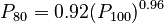$P_{80}=0.92 × (P_{100})^{0.96}$